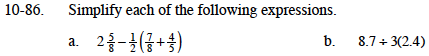### Home > MC1 > Chapter 10 > Lesson 10.2.2 > Problem10-86

10-86.Convert the first term into a fraction greater than one.

$2\frac{5}{8}=\frac{21}{8}$

$\frac{7}{8}+\frac{4}{5}=\frac{35}{40}+\frac{32}{40}=\frac{67}{40}$
$\frac{1}{2}\left(\frac{67}{40}\right)=\frac{67}{80}$
$\frac{21}{8}\minus\frac{67}{80}=\frac{210}{80}\minus\frac{67}{80}=\frac{143}{80}=1\frac{63}{80}$